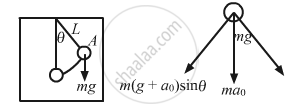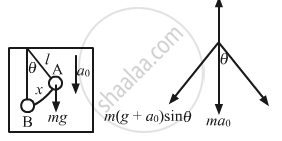Advertisement Remove all ads

# A Simple Pendulum of Length L is Suspended Through the Ceiling of an Elevator. Find the Time Period of Small Oscillations - Physics

Sum

A simple pendulum of length l is suspended through the ceiling of an elevator. Find the time period of small oscillations if the elevator (a) is going up with and acceleration a0(b) is going down with an acceleration a0 and (c) is moving with a uniform velocity.

Advertisement Remove all ads

#### Solution

The length of the simple pendulum is l.
​Let x be the displacement of the simple pendulum..
(a)From the diagram, the driving forces f is given by,
f = m(g + a0)sinθ                 ...(1)
Acceleration (a) of the elevator is given by,

$a = \frac{f}{m}$

$= \left( g + a_0 \right)\sin\theta$

$= \left( g + a_0 \right)\frac{x}{l} \left( \text{From the diagram } \sin\theta = \frac{x}{l} \right)_{}$

[ when θ is very small, sin θ → θ = x/l]

$\therefore a = \left( \frac{g + a_0}{l} \right)x$  ...(2)

As the acceleration is directly proportional to displacement, the pendulum executes S.H.M.
Comparing equation (2) with the expression a =$\omega^2 x$,we get:

$\omega^2 = \frac{g + a_0}{l}$

Thus, time period of small oscillations when elevator is going upward(T) will be:

$T = 2\pi\sqrt{\frac{l}{g + a_0}}$When the elevator moves downwards with acceleration a0,
Driving force (F) is given by,
F = m(g − a0)sinθ
On comparing the above equation with the expression, F = ma,$\text{Acceleration}, a = \left( g - a_0 \right) sin\theta = \frac{\left( g - a_0 \right)x}{l} = \omega^2 x$ $\text{Time period of elevator when it is moving downward}\left( T' \right) \text{ is given by,}$

$T' = \frac{2\pi}{\omega} = 2\pi\sqrt{\frac{l}{g - a_0}}$

(c) When the elevator moves with uniform velocity, i.e. a0 = 0,
For a simple pendulum, the driving force $\left( F \right)$ is given by,

$F = \frac{mgx}{l}$

$\text{Comparing the above equation with the expression, F = ma, we get: }$ $a = \frac{gx}{l}$ $\Rightarrow \frac{x}{a} = \frac{l}{g}$ $T = 2\pi\sqrt{\frac{\text{displacement}}{\text{Acceleration}}}$ $= 2\pi\sqrt{\frac{l}{g}}$

Is there an error in this question or solution?
Advertisement Remove all ads

#### APPEARS IN

HC Verma Class 11, 12 Concepts of Physics 1
Chapter 12 Simple Harmonics Motion
Q 43 | Page 255
Advertisement Remove all ads

#### Video TutorialsVIEW ALL 

Advertisement Remove all ads
Share
Notifications

View all notifications

Forgot password?